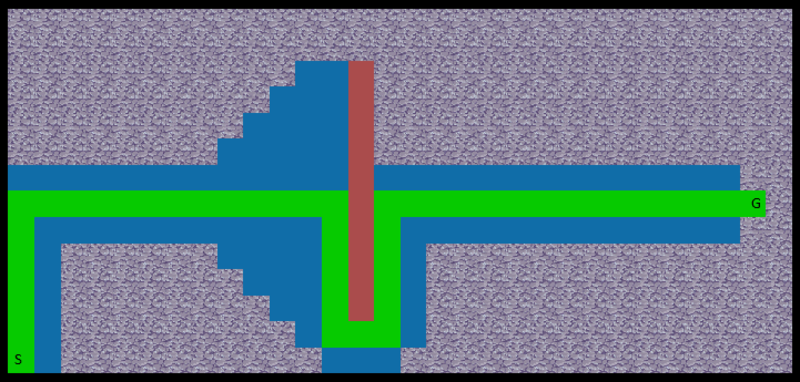2 views

edited

I'm in the process of making a 2D tile map and I'm now trying to implement A* pathfinding. I'm following the Wikipedia pseudocode for A*.

Things are going quite well except for some weird behavior in the decisions taken by the algorithm.

My code so far:

void Pathfinding(Point from, Point destination) { goalNode = new Node(destination, 0, 0); startNode = new Node(from, 0, ManhattanDistance(from, destination)); open = new List<Node>(); //list of nodes closed = new List<Node>(); open.Add(startNode); //Add starting point while(open.Count > 0) { node = getBestNode(); //Get node with lowest F value if(node.position == goalNode.position) { Debug.Log("Goal reached"); getPath(node); break; } removeNode(node, open); closed.Add(node); List<Node> neighbors = getNeighbors(node); foreach(Node n in neighbors) { float g_score = node.G + 1; float h_score = ManhattanDistance(n.position, goalNode.position); float f_score = g_score + h_score; if(isValueInList(n, closed) && f_score >= n.F) continue; if(!isValueInList(n, open) || f_score < n.F) { n.parent = node; n.G = g_score; n.G = h_score; if(!isValueInList(n, open)) { map_data[n.position.x, n.position.y] = 4; open.Add(n); } } } } }

The result of running this code:Blue is the nodes from the open list and green is the path chosen to the goal node.

SOLUTION:

void Pathfinding(Point from, Point destination) { goalNode = new Node(destination, 0, 0); startNode = new Node(from, 0, ManhattanDistance(from, destination)); open = new List<Node>(); //list of nodes closed = new List<Node>(); open.Add(startNode); //Add starting point while(open.Count > 0) { node = getBestNode(); //Get node with lowest F value if(node.position == goalNode.position) { Debug.Log("Goal reached"); getPath(node); break; } removeNode(node, open); closed.Add(node); List<Node> neighbors = getNeighbors(node); foreach(Node n in neighbors) { float g_score = node.G + 1; float h_score = ManhattanDistance(n.position, goalNode.position); float f_score = g_score + h_score; if(isValueInList(n, closed) && f_score >= n.F) continue; if(!isValueInList(n, open) || f_score < n.F) { n.parent = node; n.G = g_score; n.H = h_score; if(!isValueInList(n, open)) { map_data[n.position.x, n.position.y] = 4; open.Add(n); } } } } }

by (108k points)

Firstly, your open nodes must be sorted in descending order, while in your code there is no order. You compute the distance (g) and the heuristics (h) but never actually use it. You should consider using ordered container instead of lists (as sorting lists in each iteration won't be efficient)

And secondly, you have not stored the heuristic value in the node as

n.G = h_score;

it should be like this:

n.H = h_score;